NAND, NOR & XOR Logic Gates

Lesson Transcript
Instructor: Rocco Arizzi

Rocco has a PhD. in Electrical Engineering, a graduate certificate in Cybersecurity, and has taught university Math, Physics, Engineering, and Computer Science.

In electronics, logic gates operate basic functions, providing a foundation for digital circuits. Learn about NAND, NOR, and XOR logic gates. Explore logic truth tables, functional completeness, and Boolean logic. Updated: 01/23/2022

Logic Truth Tables & Logic Gates

The three basic Boolean logic operations of AND, OR, and NOT are the most familiar in human language and the most intuitive to understand. To a computer, however, they are just a collection of binary truth tables. For a general logic gate with two inputs and a single output, there are 16 possible outcomes (2^4), two of which are covered by the AND and OR gates. The single-input NOT gate adds a 17th outcome. Figure 1 reviews the truth tables that correspond to the AND, OR, and NOT gates.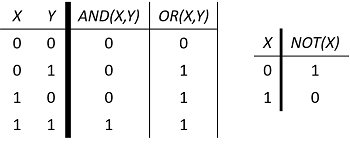It is useful in practical digital systems design to have more truth table options to choose from. The NAND, NOR, and XOR gates add additional diversity to the set of binary truth tables and each have their own useful properties.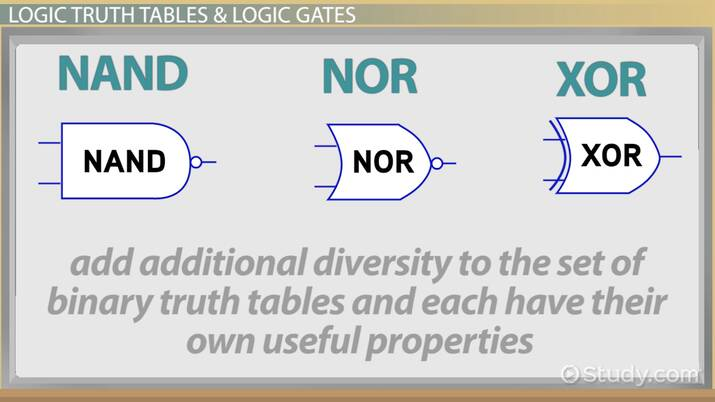An error occurred trying to load this video.

Try refreshing the page, or contact customer support.

Coming up next: How to Simplify & Combine Logic Circuits

You're on a roll. Keep up the good work!

Replay
Your next lesson will play in 10 seconds
• 0:04 Logic Truth Tables &…
• 0:53 NAND, NOR, & XOR Logic Gates
• 2:07 Functional Completeness
• 3:22 Lesson Summary
Save Save

Want to watch this again later?

Timeline
Autoplay
Autoplay
Speed Speed

NAND, NOR, and XOR Logic Gates

The negated AND operation, or NAND gate, is the binary complement of an AND operation:

NAND(X,Y) = NOT(AND(X,Y))

Since the NOT operation is invertible, this relationship can also be written as:

AND(X,Y) = NOT(NAND(X,Y))

Similarly, the NOR gate is defined as a negated OR operation:

NOR(X,Y) = NOT(OR(X,Y))

OR can also be expressed in terms of NOR:

OR(X,Y) = NOT(NOR(X,Y))

The definition of the OR operation is that it is TRUE if either input is TRUE. This includes the possibility that both inputs are TRUE. In contrast, the exclusive OR operation or XOR (sometimes called EOR) gate is TRUE if and only if a single input is true.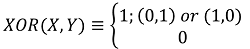This relationship has two equally correct expressions in terms of the basic logic gates:

XOR(X,Y) = OR(AND(X, NOT(Y)),AND(NOT(X),Y)) = AND(OR(X,Y), OR(NOT(X), NOT(Y)))

The truth tables and distinctive shape set symbols are given for NAND, NOR, and XOR in Figure 2. It is readily evident that the outputs of NAND and NOR are the exact complement of AND and OR, respectively.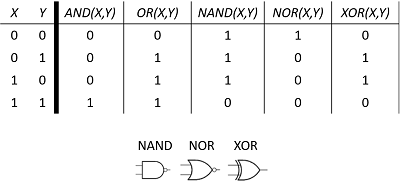Functional Completeness

If the two inputs to a NAND gate are shorted together, as shown in Figure 3, the only relevant portions of the truth table are the ones for which the two inputs are equal.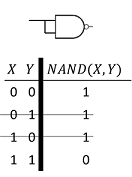To unlock this lesson you must be a Study.com Member.

Register to view this lesson

Are you a student or a teacher?